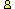CTK Exchange

 Subject: "Problem with the Floor van Lamoen Pythagorean Proof" Previous Topic | Next Topic
 ConferencesThe CTK ExchangeGuest bookTopic #530Printer-friendly copyEmail this topic to a friend Reading Topic #530
reb_sab1492
Member since May-2-06
May-02-06, 02:02 PM (EST)"Problem with the Floor van Lamoen Pythagorean Proof"

 Okay, so this is an example of the proof i found on a site:This is another proof by Floor van Lamoen; Floor has been led to the proof via Bottema's theorem. However, the theorem is not actually needed to carry out the proof. In the figure, M is the center of square ABA'B'. Triangle AB'C' is a rotation of triangle ABC. So we see that B' lies on C'B''. Similarly, A' lies on A''C''. Both AA'' and BB'' equal a + b. Thus the distance from M to AC' as well as to B'C' is equal to (a + b)/2. This gives Area(AMB'C') = Area(MAC') + Area(MBC') = (a + b)/2 · b/2 + (a + b)/2 · a/2 = a2/4 + ab/2 + b2/4. But also: Area(AMB'C') = Area(AMB') + Area(ABC') = c2/4 + ab/2. This yields a2/4 + b2/4 = c2/4 and the Pythagorean theorem.our problem is with the first area equationArea(AMB'C') = Area(MAC') + Area(MBC') = (a + b)/2 · b/2 + (a + b)/2 · a/2 = a2/4 + ab/2 + b2/4. we can see which triangles they are most likely talking about, but they would be MAC' and MB'C' or MBC" So if anyone could please clarify this and possibly explain where the second part of the equation is coming from it would be a great help.Since i was unable to upload the picture here, i am including a link of the site i found it onhttps://www.cut-the-knot.org/pythagoras/index.shtmlit's proof #63Thanks!Reb and Sab

alexbCharter Member
1828 posts
May-02-06, 02:15 PM (EST)1. "RE: Problem with the Floor van Lamoen Pythagorean Proof"
In response to message #0

 There have been two typos. Many thanks for bringing this up.Area(AMB'C') = Area(MAC') + Area(MBC') should have beenArea(AMB'C') = Area(MAC') + Area(MB'C') andArea(AMB'C') = Area(AMB') + Area(ABC')should have beenArea(AMB'C') = Area(AMB') + Area(AB'C')Both are fixed now.

 Conferences | Forums | Topics | Previous Topic | Next Topic
 Select another forum or conference Lobby The CTK Exchange (Conference)   |--Early math (Public)   |--Middle school (Public)   |--High school (Public)   |--College math (Public)   |--This and that (Public)   |--Guest book (Public) Educational Press (Conference)   |--No Child Left Behind (Public)   |--Math Wars (Public)   |--Mathematics and general education (Public)You may be curious to visit the old Guest book.